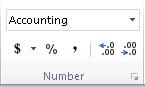Subscribe

# Making sense of dollar signs in Excel

We’ve noticed some of you searching for help using “\$” – a dollar sign. In Excel, a dollar sign can denote a currency format, but it has another common use: indicating absolute cell references in formulas. Let’s consider both uses of the dollar sign in Excel.## Microsoft Excel

Turn data into insights.

## Dollar signs denoting currency

If you want to display numbers as monetary values, you must format those numbers as currency. To do this, you apply either the Currency or Accounting number format to the cells that you want to format. The number formatting options are available on the Home tab, in the Number group.What’s the difference between the two number formats? There are two main differences:

1. The Currency format displays the currency symbol adjacent to the number, whereas the Accounting format displays the symbol at the edge of the cell, regardless of the length of the number.
2. The Accounting format displays zeros as dashes and negative numbers in parentheses, whereas the Currency format displays zeros as zeros and denotes negative numbers by using a minus sign (-). For more information, see the article Display numbers as currency.

## Dollar signs indicating absolute references

You probably know that a formula can refer to cells. That’s one reason Excel formulas are so powerful — the results can change based on changes made in other cells. When a formula refers to a cell, it uses a cell reference. In the “A1” reference style (the default), there are three kinds of cell references: absolute, relative, and mixed.

### Absolute cell references

When a formula contains an absolute reference, no matter which cell the formula occupies the cell reference does not change: if you copy or move the formula, it refers to the same cell as it did in its original location. In an absolute reference, each part of the reference (the letter that refers to the row and the number that refers to the column) is preceded by a “\$” – for example, \$A\$1 is an absolute reference to cell A1. Wherever the formula is copied or moved, it always refers to cell A1.

### Relative cell references

In contrast, a relative reference changes if the formula is copied or moved to a different cell (i.e., a cell other than where the formula was originally entered). The row and column portions of a relative reference are not preceded by a “\$” –  for example, A1 is a relative reference to cell A1. If moved or copied, the reference changes by the same number of rows and coulmns as it was moved. So, if you move a formula with the relative reference A1 one cell down and one cell to the right, the reference changes to B2.

### Mixed cell references

A mixed reference uses a dollar sign either in front of the row letter or in front of the column number, but not both – for example, A\$1 is a mixed reference in which the row adjusts, but the column does not. So if you move a formula containing that reference one cell down and one cell to the right, it becomes B\$1.

### Which kind of cell reference should you use?

The kind of cell reference you use depends on what you are doing, but usually you want to use relative references. Excel uses relative references by default, which makes it easy to fill formulas down and across: the references automatically update, which is what you want, most of the time.

One case where you might want to use an absolute reference is when using the VLOOKUP function. Though the value you’re wanting to look up might change (for example, as you fill the VLOOKUP formula down a column), the actual location of the lookup table doesn’t change or adjust as you fill the column down.

But now that you know the differences between these kinds of cell references, you can make the decision for yourself, based on how you want your formulas to behave. Do you have some use-cases to share with the community? Share them by leaving a comment!

— Steven Thomas